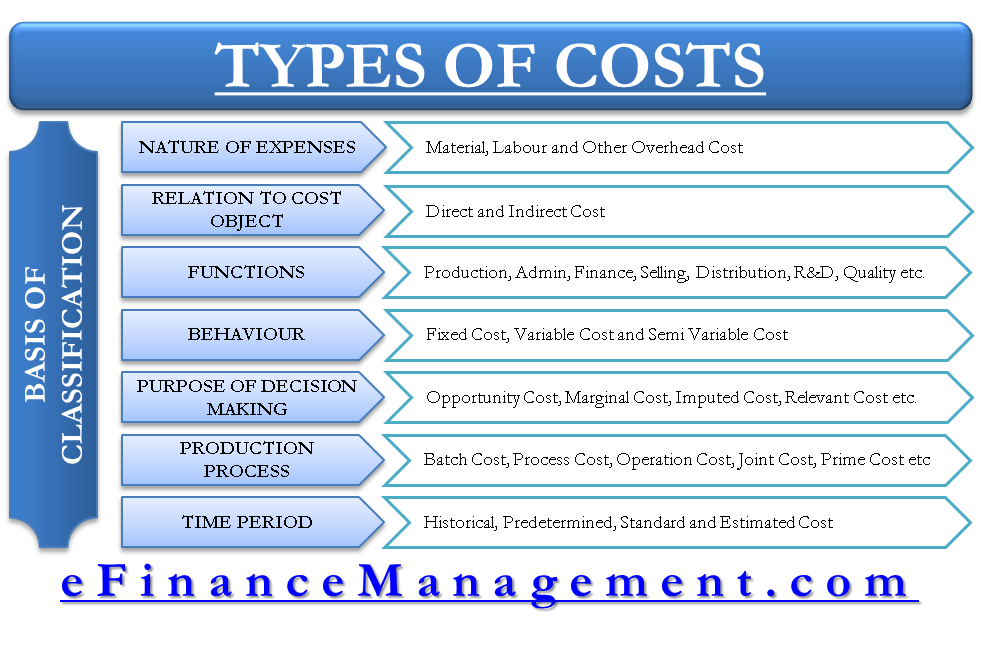# Types of Costs and their Basis of Classification

Cost classification can be done in various ways depending on its nature and a specific purpose. There are various types of costs classified into logical groupings. These groups are such that each and every item of cost can be classified. These classifications of costs make the cost information meaningful. It is of utmost importance to the management of a manufacturing concern. It is the first step towards their decision-making process relating to costs and costing.

Cost is a very vague term and barely has any meaning until it is classified into logical groups. For example, if we know total expenses are of \$500 Million against \$550 Million revenue.What insight can we derive? Nothing. Now if we add one more information say \$350 Million is direct material cost and \$100 Million is a direct labor and other overheads. Now, the management can focus on direct material cost because that forms the major part of the total cost as far as cost control initiatives are concerned.## Basis of Classification of Cost

There can be various bases on which classification of costs can be done. Following are the important ones, we will discuss in brief.

1. Nature of Expense: By nature of expenses, costs are classified into material, labor and expenses.

2. Relation to Cost Object – Trace-ability: This classification is based on the relation of cost element with the cost object. The classification is done into direct and indirect costs. The basis is cause and effect relationship between cost element and cost object or trace-ability of costs to its cost object.

3. Functions / Activities: Costs can also be classified into various functions / activities. Common functional classification of costs are done into following:

• Production
• Finance
• Selling
• Distribution
• Research and Development
• Quality Check etc.

4. The behavior of Costs: The behavior of costs is seen with respect to the change in volume. On this basis, costs are classified into Fixed Costs, Variable Costs, and Semi-variable Costs. Fixed costs do not change with the change in volume, variable costs do change. Semi-variable costs does not change up to a particular level of activity, beyond that it will change.

5. The purpose of Decision Making by Management: For decision-making purpose of management, costs can be classified into various types such as opportunity cost, marginal cost, differential cost, relevant cost, imputed cost, replacement cost, sunk cost, normal/ abnormal cost, avoidable/ unavoidable costs, etc.

6. Production Process: It’s an important classification for cost accounting of different manufacturing industries. Based on the production process of the industry, costs can be classified into following:

• Batch Cost
• Process Cost
• Operation Cost
• Operating Cost
• Contract Cost
• Joint Cost
• Prime Cost
• Factory Cost or Works Cost
• Cost of Production
• Cost of Goods Sold

7. Time Period: Based on a time period of assessment or any other specific purpose, costs can be classified into historical cost, pre-determined cost, standard cost, and estimated cost.

Last updated on : March 23rd, 2019
1.Simon mwangi
2.Ezinne
3.Dee
4.ROBERT